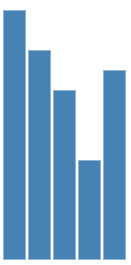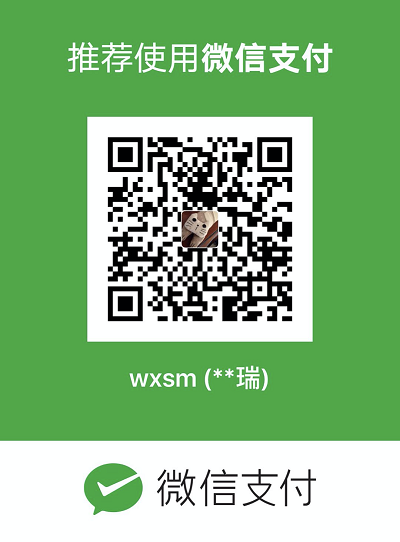D3 (Data-Driven Documents) 是一个 JavaScript Library，用来做 Web 端的数据可视化实现以及各种绘图。

D3.js is a JavaScript library for manipulating documents based on data. D3 helps you bring data to life using HTML, SVG, and CSS.

1. HTML / DOM
2. CSS
3. JavaScript (better with jQuery)
4. SVG

HTML / CSS 不必多说，因为 D3 含有大量链式操作函数以及选择器等，因此如果有 jQuery 基础将轻松很多。此外，由于一般采用 SVG 方式进行绘图，所以 SVG 基础知识也需要掌握。

## 关于 SVG

SVG (Scalable Vector Graphics) 是一种绘图标准，已经被绝大多数的现代浏览器所支持。SVG 采用 XML 语法定义图像，可直接嵌入 HTML 中使用。

SVG 的特点是矢量绘图（与 Canvas 不同），除了预设样式以外同时也支持 CSS 样式。

``````<svg>
<circle cx="100" cy="50" r="40" stroke="black" stroke-width="2" fill="red"/>
</svg>``````

SVG 有一些预定义的形状元素，可被开发者使用和操作：

• 矩形 `<rect>`
• 圆形 `<circle>`
• 椭圆 `<ellipse>`
• 线 `<line>`
• 折线 `<polyline>`
• 多边形 `<polygon>`
• 路径 `<path>`

## 选择器

### 选择元素

D3 使用与 jQuery 类似的选择器来获取 HTML 元素。常用的方法有：

• `d3.select(selector)`
• `d3.selectAll(selector)`

（参数既可以传 selector 也可以直接传 HTML Element ）

``````d3.select('body') //选择 body 元素

d3.selectAll('p') //选择所有 p 元素

d3.selectAll('.className') //选择所有 class 包含 className 的元素``````

### 操作选择

• 在此选择的基础上继续选择；
• 改变属性；
• 改变样式；
• 绑定事件；
• 插入、删除；
• 绑定数据。

## 数据绑定

• `datum`: 将一个数据绑定到选择上；
• `data`: 将一个数组绑定到选择上，数组的各项分别与选择的各项一一对应。

``````<p>Apple</p>
<p>Pear</p>
<p>Banana</p>``````

### datum

``````let str = 'datum';
let p = d3.selectAll('p');

p.datum(str);
p.text((d, i) => `Element \${i} bind with \${d}`);``````

``````Element 0 bind with datum
Element 1 bind with datum
Element 2 bind with datum``````

### data

``````let strArr = ['data0', 'data1', 'data2'];
let p = d3.selectAll('p');

p.data(strArr);
p.text((d, i) => `Element \${i} bind with \${d}`);``````

``````Element 0 bind with data0
Element 1 bind with data1
Element 2 bind with data2``````

## 实践：简单柱状图

``````let width = 300,
height = 300；

let svg = d3.select('body')
.append('svg')
.attr('width', width)
.attr('height', height);``````

``let data = [250, 210, 170, 100, 190];``

``````let rectWidth = 25;

svg.selectAll('rect')
.data(data)
.enter()
.append('rect')
.attr('y', (d, i) => height - d)
.attr('x', (d, i) => i * rectWidth)
.attr('height', d => d)
.attr('width', rectWidth - 2)
.attr('fill', 'steelblue');``````

`rectWidth` 表示柱子的宽度，至于坐标、宽高则分别通过 x / y 以及 height / width 属性来控制，效果如下：微信支付宝